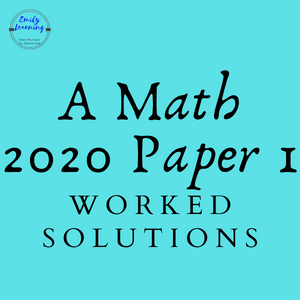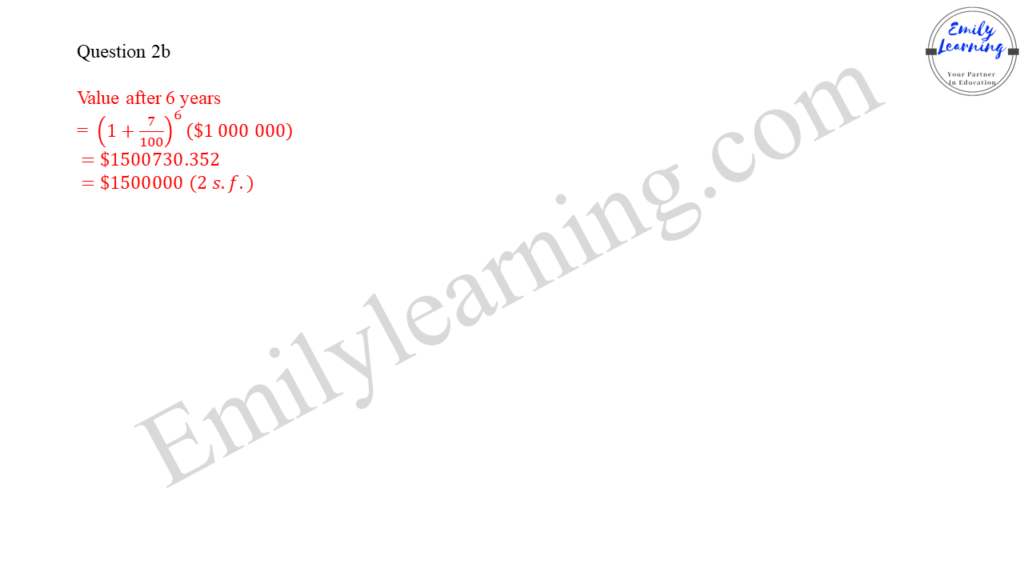# Suggested Solutions: 2020 GCE O Level A Math Paper 1Here, you will find the suggested answer for 2020 GCE O Level A Math exam. Click on the link below to go to the question directly.

Question 1 (Note: No longer tested in O Level A Math from 2021 onwards: sum and product of roots of quadratic functions)

Question 2

Question 3

Question 4

Question 5 (Note: Absolute functions are no longer tested in O Level A Math from 2021 onwards)

Question 6

Question 7

Question 8

Question 9

Question 10

Question 11

Question 12

## Question 1 on Quadratic Functions

This question on sum of roots and product of roots of a quadratic equation is no longer tested from 2021 onwards. I’ve still included the solutions for this question, if you are interested.

## Question 2 on Indices

This is a question on indices and surds. In part (a), students are tested on the laws of indices.

In part (b), it’s quite straight forward. Sort of like compound interest which you have learnt in elementary Math.worked solutions of O Level A Math Paper 1 question 2 part b on indices and surds

## Question 3 on Partial Fractions

This question is on partial fractions. An improper fraction is given, hence students are required to make it a proper fraction in addition to applying partial fractions.

## Question 4 on Application of Differentiation – Increasing function

In this question, students need to apply the concept that when a function is increasing, dy/dx > 0.

## Question 5 on Absolute Functions or | |

Absolute functions are no longer tested in GCE O Level for A Math from 2021 onwards. I have included the solutions for this, just in case you are interested.

## Question 6 on Application of differentiation – finding stationary points

In this question, students are expected to use differentiation to find stationary points.

## Question 7 on Trigonometry (solving equations and applying identities)

In this question, students need to make use of trigonometric identities to manipulate the given trigonometry equation. There after, they then solve the trigonometric equation

## Question 8 on Polynomials – Factor Remainder Theorem and solving cubic equations

There are 2 parts in this question.

In part a, students use the concept learnt in the chapter on factor- remainder theorem.

In part b, students are asked to solve cubic equations.

## Question 9 on Coordinate Geometry and Circles

This is a coordinate geometry question, with the main focus on circles in coordinate geometry.

## Question 10 on Finding Area by Integration

This is a question involving finding area between two graphs by integration.

## Question 11 on Application of Integration and Differentiation to Kinematics

This is a question on kinematics. Students are required to find distance and acceleration given velocity.

To find distance, you’ll need to integration velocity.

To find acceleration, differentiate velocity.

## Question 12 on Trigonometry

This is a long question on trigonometry with 6 parts! The question mainly revolve around using of identities, and knowing the sign of the trigonometric ratios in different quadrants (recall ASTC).

## Learn On-demand – A- Math Courses

If you want to learn a particular topic in detail, check out our on-demand O Level A Math courses here.

Suggested Answers for other A Math Papers: